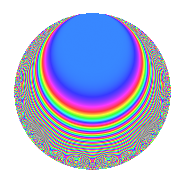# Properties

 Label 62.2.gLevel 62 Weight 2 Character orbit g Rep. character $$\chi_{62}(7,\cdot)$$ Character field $$\Q(\zeta_{15})$$ Dimension 16 Newforms 2 Sturm bound 16 Trace bound 2

# Related objects

## Defining parameters

 Level: $$N$$ = $$62 = 2 \cdot 31$$ Weight: $$k$$ = $$2$$ Character orbit: $$[\chi]$$ = 62.g (of order $$15$$ and degree $$8$$) Character conductor: $$\operatorname{cond}(\chi)$$ = $$31$$ Character field: $$\Q(\zeta_{15})$$ Newforms: $$2$$ Sturm bound: $$16$$ Trace bound: $$2$$ Distinguishing $$T_p$$: $$3$$

## Dimensions

The following table gives the dimensions of various subspaces of $$M_{2}(62, [\chi])$$.

Total New Old
Modular forms 80 16 64
Cusp forms 48 16 32
Eisenstein series 32 0 32

## Trace form

 $$16q + 4q^{3} - 4q^{4} - 2q^{5} - 8q^{6} - 14q^{7} + 4q^{9} + O(q^{10})$$ $$16q + 4q^{3} - 4q^{4} - 2q^{5} - 8q^{6} - 14q^{7} + 4q^{9} + 4q^{10} - 10q^{11} + 4q^{12} - 12q^{14} - 10q^{15} - 4q^{16} - 4q^{17} + 8q^{18} - 2q^{20} - 30q^{21} + 14q^{22} + 18q^{23} + 2q^{24} + 10q^{25} - 20q^{26} - 8q^{27} + 16q^{28} + 24q^{29} + 28q^{30} - 8q^{33} + 40q^{34} + 40q^{35} + 4q^{36} + 14q^{37} - 6q^{38} - 20q^{39} - 6q^{40} - 6q^{41} + 12q^{42} - 26q^{43} + 2q^{45} - 12q^{46} + 10q^{47} - 6q^{48} - 14q^{49} - 28q^{50} + 12q^{51} - 34q^{53} - 28q^{54} + 8q^{55} - 2q^{56} + 24q^{57} - 12q^{58} - 10q^{59} - 10q^{60} + 28q^{61} - 38q^{62} - 28q^{63} - 4q^{64} - 20q^{65} - 16q^{66} - 6q^{67} - 14q^{68} + 28q^{69} - 8q^{70} + 16q^{71} + 8q^{72} - 46q^{73} - 2q^{74} - 18q^{75} - 30q^{76} - 2q^{77} - 20q^{78} - 10q^{79} + 18q^{80} + 28q^{82} + 46q^{83} + 30q^{84} + 12q^{85} + 34q^{86} + 12q^{87} + 4q^{88} + 36q^{89} + 30q^{90} + 40q^{91} + 8q^{92} + 76q^{93} + 32q^{94} + 54q^{95} + 2q^{96} - 22q^{97} + 28q^{98} - 4q^{99} + O(q^{100})$$

## Decomposition of $$S_{2}^{\mathrm{new}}(62, [\chi])$$ into irreducible Hecke orbits

Label Dim. $$A$$ Field CM Traces $q$-expansion
$$a_2$$ $$a_3$$ $$a_5$$ $$a_7$$
62.2.g.a $$8$$ $$0.495$$ $$\Q(\zeta_{15})$$ None $$-2$$ $$3$$ $$1$$ $$2$$ $$q+\zeta_{15}^{6}q^{2}+(\zeta_{15}+\zeta_{15}^{4}+\zeta_{15}^{7})q^{3}+\cdots$$
62.2.g.b $$8$$ $$0.495$$ $$\Q(\zeta_{15})$$ None $$2$$ $$1$$ $$-3$$ $$-16$$ $$q-\zeta_{15}^{6}q^{2}+(\zeta_{15}-\zeta_{15}^{4}+\zeta_{15}^{7})q^{3}+\cdots$$

## Decomposition of $$S_{2}^{\mathrm{old}}(62, [\chi])$$ into lower level spaces

$$S_{2}^{\mathrm{old}}(62, [\chi]) \cong$$ $$S_{2}^{\mathrm{new}}(31, [\chi])$$$$^{\oplus 2}$$